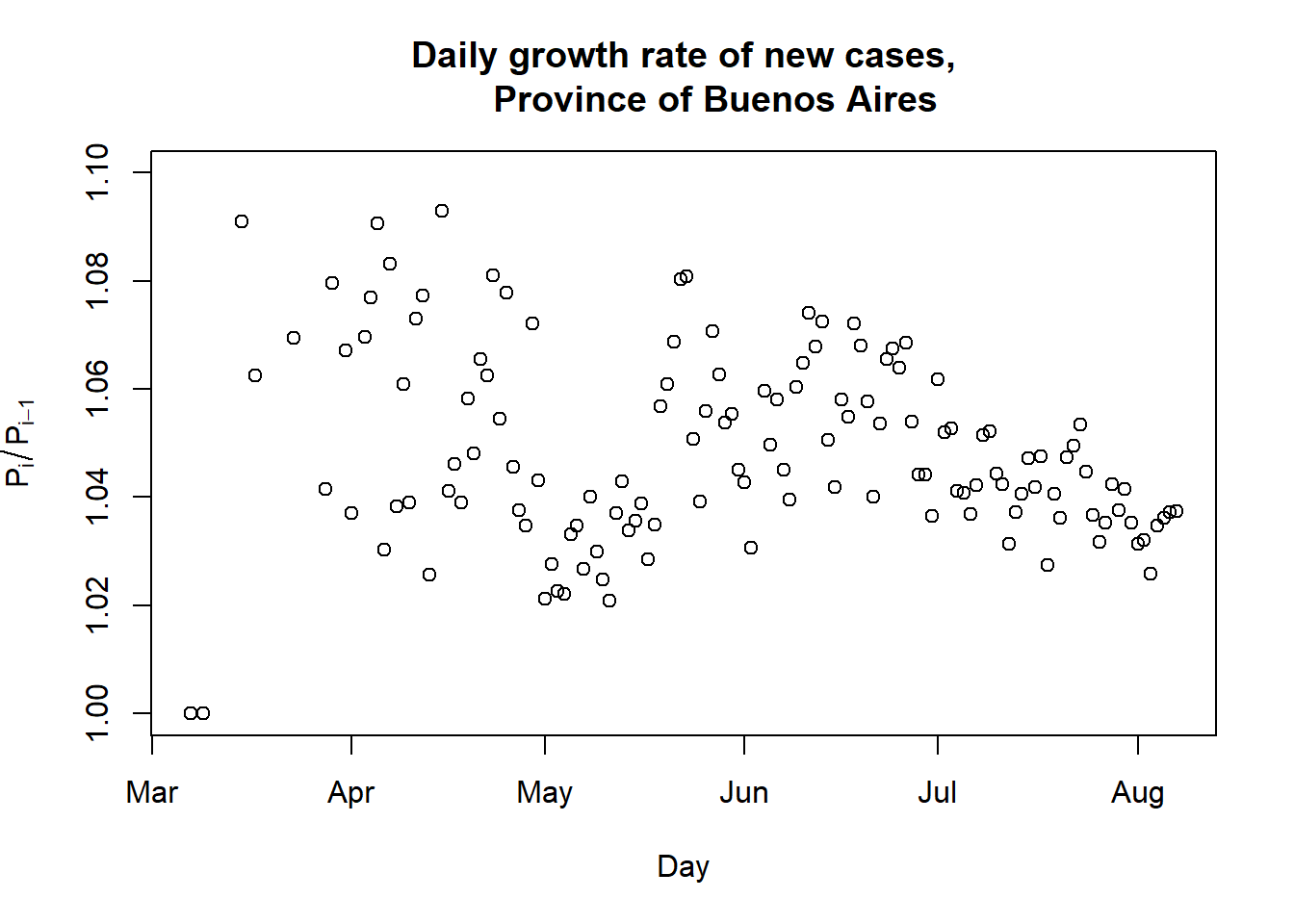# ¿What is herd immunity?

Sometimes we hear media talk about “the controversial theory of herd immunity”, as if herd immunity was not an established fact. The problem is that herd immunity as a fact is confused with seeking herd immunity as a public-health strategy, which are two different things. Herd immunity exists and is given by a simple formula: $$1-1/R_0$$. (Where this formula comes from is explained here.)

This formula tells us that for a given $$R_0$$ (greater than 1), herd immunity is attained when $$(1-1/R_0)\times 100\%$$ of the population has been infected. After that, the daily number of new infections begins to go down (as long as $$R_0$$ stays at the same level).

Then there are two scenarios for the evolution of epidemics. Scenario A is when a value of $$R_0$$ less than 1 is attained: in that case the curve of new daily infections always goes down, regardless of the total number of infecteds. Scenario B is when $$R_0$$ is bigger than 1: in that case the daily number of infections begins to go down only after $$(1-1/R_0)\times 100\%$$ of the population has been infected.

The following table shows some values of $$R_0$$ and the corresponding $$(1-1/R_0)\times 100\%$$, just to give an idea.

$$R_0$$ Percentage of infecteds for herd immunity
1.02 2 %
1.04 4 %
1.06 6 %
1.08 7 %
1.10 9 %
1.15 13 %
1.20 17 %
1.25 20 %

So, for example, if a city can keep $$R_0$$ at 1.04, it is necessary that 4% of the population get infected to start to see a decrease in new daily infections.

# Examples

## City of Buenos Aires

Computing $$R_0$$ is complicated because the SIRD model must be fit to the data. In this post it is estimated that $$R_0$$ is between 1.04 and 1.10 for the city of Buenos Aires, so herd immunity will be attained when between 4% and 9% of the population gets infected. To date, the city reports 102,638 confirmed cases, which represents 3,5% of the population of 2,890,000. So, for an $$R_0$$ of 1.04 herd immunity has almost been attained in the city, and the number of new daily infections should start to go down soon.

Of course, as people’s mobility grows, $$R_0$$ will also grow, so, if $$R_0$$ goes up to 1.10, say, then there is going to be a “flare-up” that raises the number of total cases to 9% of the population, or 260,000 cases. And so on, as mobility and $$R_0$$ keep growing.

(Note: the actual number of cases is higher than the number of confirmed cases, maybe 10 times higher, but then also the true $$R_0$$ is higher than 1.04, so, in the end, both effects more or less cancel each other and the conclusions are the same whether you consider confirmed cases or actual cases.)

## Province of Buenos Aires

For the Province of Buenos Aires we take only the 10,894,664 inhabitants of the City of Buenos Aires’ suburbs as population, because in the rest of the province the cases are relatively few, the towns are more isolated and the virus does not circulate as easily as in Buenos Aires’ suburbs.

Then $$R_0$$ has also hovered around 1.04 in the province. The corresponding 4% herd immunity will then be attained with 436,000 confirmed cases. But the total number of confirmed cases so far is only 144,307, or 1.3% of the population, so the peak is still in the future in the province.

¿When will the peak be reached in the province, then? To get an idea of the growth rate of new cases, we can graph the ratio of total cases each day over the total cases for the previous day, which gives the daily growth rate:The average daily growth rate in the last 7 days was 3.35%. At that speed, the 436,000 total cases will be reached in 34 days, so the peak will occur in a month or so in the Province of Buenos Aires.

Back to blog